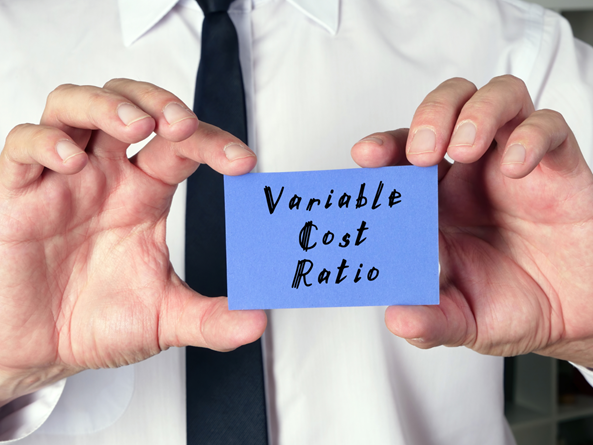# Variable Cost Ratio

• Updated on

## What is the variable cost ratio?

Variable cost ratio can be defined as the ratio between the increasing production quantity and the increasing revenue earned by a company while executing its operational activities. It is a method of measuring the dependency of variable production and a company's net income earned through production. The variable cost ratio portrays the dependency of net revenue on the variable production.

As the earning revenue directly depends upon the volume of production, we should also keep in mind that variable cost also increases with the increase in production. The variable cost ratio can be easily obtained by dividing variable cost by net sales.

Summary
• Variable cost ratio can be defined as the ratio between the increasing production quantity and the increasing revenue earned by a company while executing its operational activities.
• The variable cost ratio can be easily obtained by dividing variable cost by net sales.
• The aim of obtaining a variable cost ratio is to analyse whether the company can balance its variable cost and revenue together or not.Image source: © Rummess | Megapixl.com

## How can the concept of variable cost ratio be explained?

Variable cost ratio is a general term in every business organisation. The aim of obtaining a variable cost ratio is to analyse whether the company can balance its variable cost and revenue together or not. It is measured to ensure that the variable cost does not exceed the net revenue earned by a company.

Variable cost ratio also plays a significant role in a company's management and decision making. The profit margins and any other type of decisions related to the production and pricing of a product are measured by the variable cost ratio.

A higher amount of  fixed cost indicates a lower variable cost ratio. In that case, the company must strategically determine the appropriate selling price of a product to cover all the expenses, earn revenue and continue a sustainable business.

## How can variable cost and fixed cost be defined?

• Fixed Cost

Fixed cost refers to the cost which does not fluctuate with the change in the units of goods and services sold and produced, it is the expenses of an enterprise which have to be paid on particular time. Some common examples of fixed cost are payroll or salaries of employees, property rent, insurance coverage, property tax, etc.Image source: © Designer491 | Megapixl.com

• Variable cost

Variable cost in a business organisation can be identified by the cost incurred by a company that depends upon the production quantity. The variable cost increases with the increase in the quantity of production. It affects the net revenue earned by a company. Some common examples of variable cost are the fluctuating cost of raw materials and packaging, labour charge, which is dependent on the number of products manufactured, etc.

## How to calculate the variable cost ratio?

The variable cost ratio is calculated to portray the dependency of net revenue on the production quantity. The formula of variable cost ratio is:There is another formula to calculate the variable cost ratio.Contribution margin can be defined as the increment in the revenue earned upon selling a unit product after subtracting the variable cost.

For example:

Let us assume that the variable cost of a product is AU\$ 20 and the net revenue earned on that product is AU\$ 200. By following the first formula, the can obtain the variable cost ratio as 0.2 or 20%.

## Why is the variable cost ratio significant?

The variable cost ratio is a crucial measurement for every business organisation as it is the ideal way to analyse a company's profitability. It also assists the analysts to understand the balance between expenditure and revenue. As the variable cost ratio determines the ratio of increment in revenue with the sale of each product, it helps to understand whether a company can maintain a balance between the variable cost and revenue. The variable cost ratio also essentially helps to set the correct profit margin and determine the appropriate pricing of a product that covers both fixed and variable cost involved with its production. A higher amount of variable cost indicates that the company incurs a very less fixed cost over a period of time, say a month.

The variable cost ratio is an important financial measurement in the business world that helps the business maintain its sustainability. The management can revise the profit margin and pricing of a product at the very moment when there is an imbalance between the variable cost and net revenue.

Example of variable cost ratio:

Let us assume that company A conducts quarterly meetings to have an overview of the company performance. An important aspect of the meeting is the sales and the total revenue earned in the last quarter. This is when the variable cost ratio is essential for the company management. It helps them analyse whether the revenue and variable cost are in balance or how much revenue is needed to cover up the variable cost. The management will utilise the variable cost ratio to modify their business strategies accordingly in future.

##### Top ASX Listed Companies

Definition:
We use cookies to ensure that we give you the best experience on our website. If you continue to use this site we will assume that you are happy with it. OK